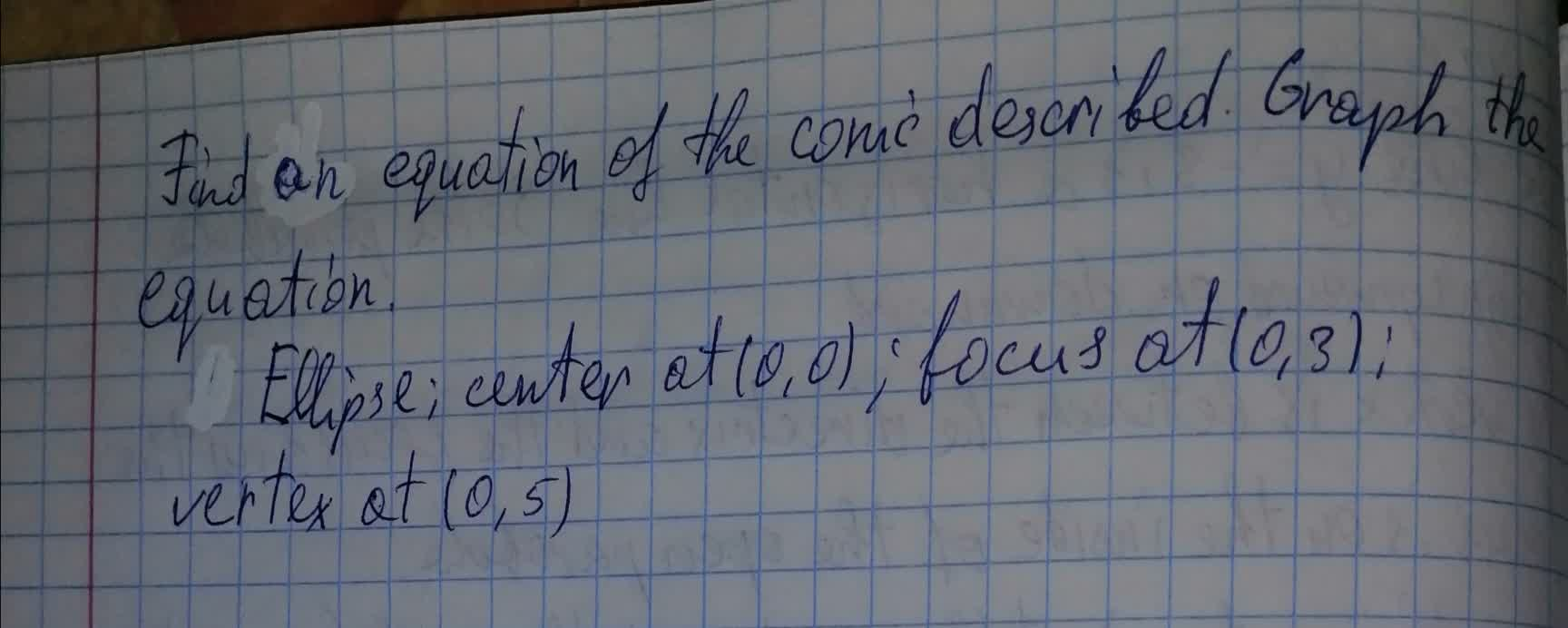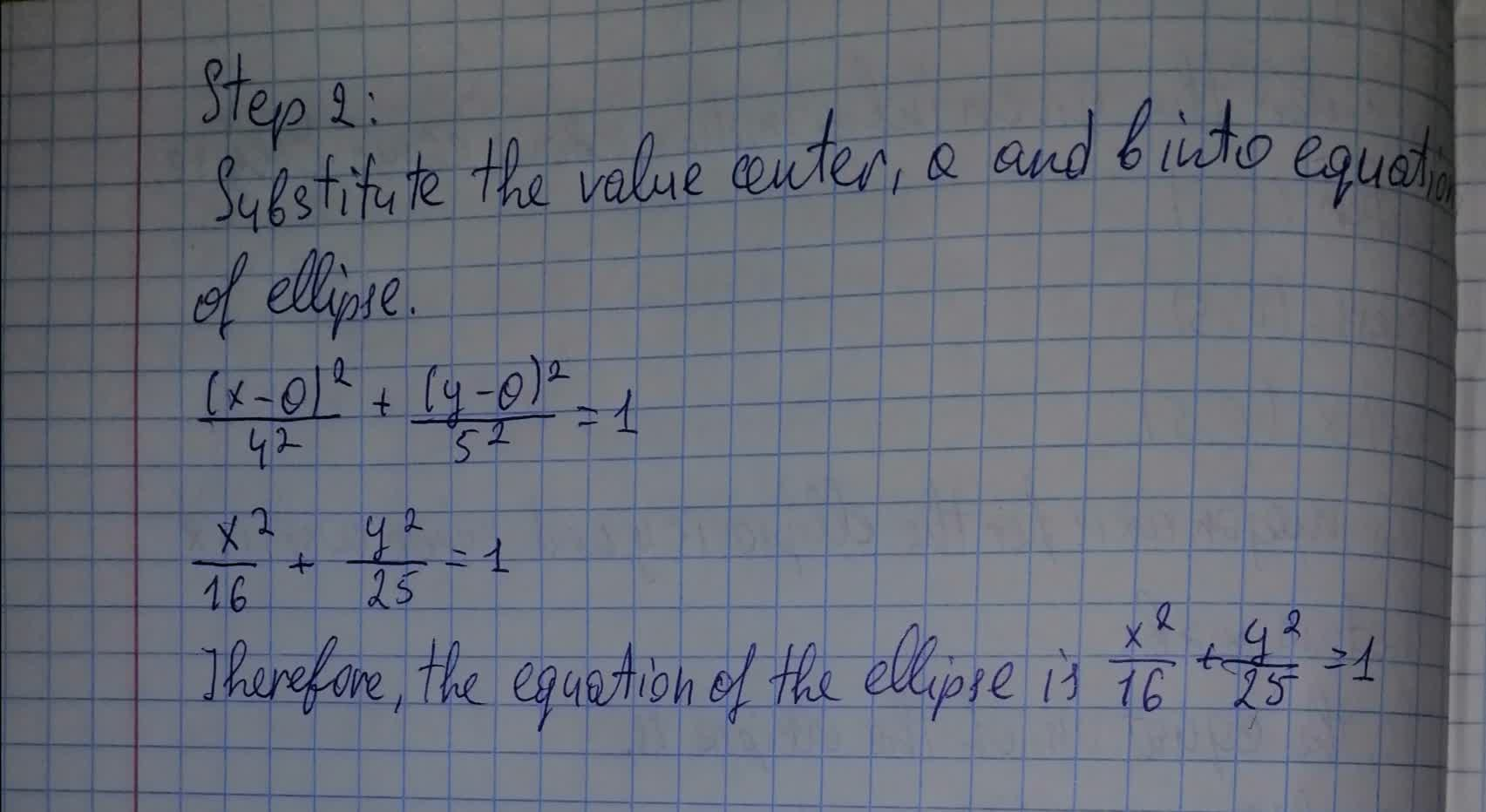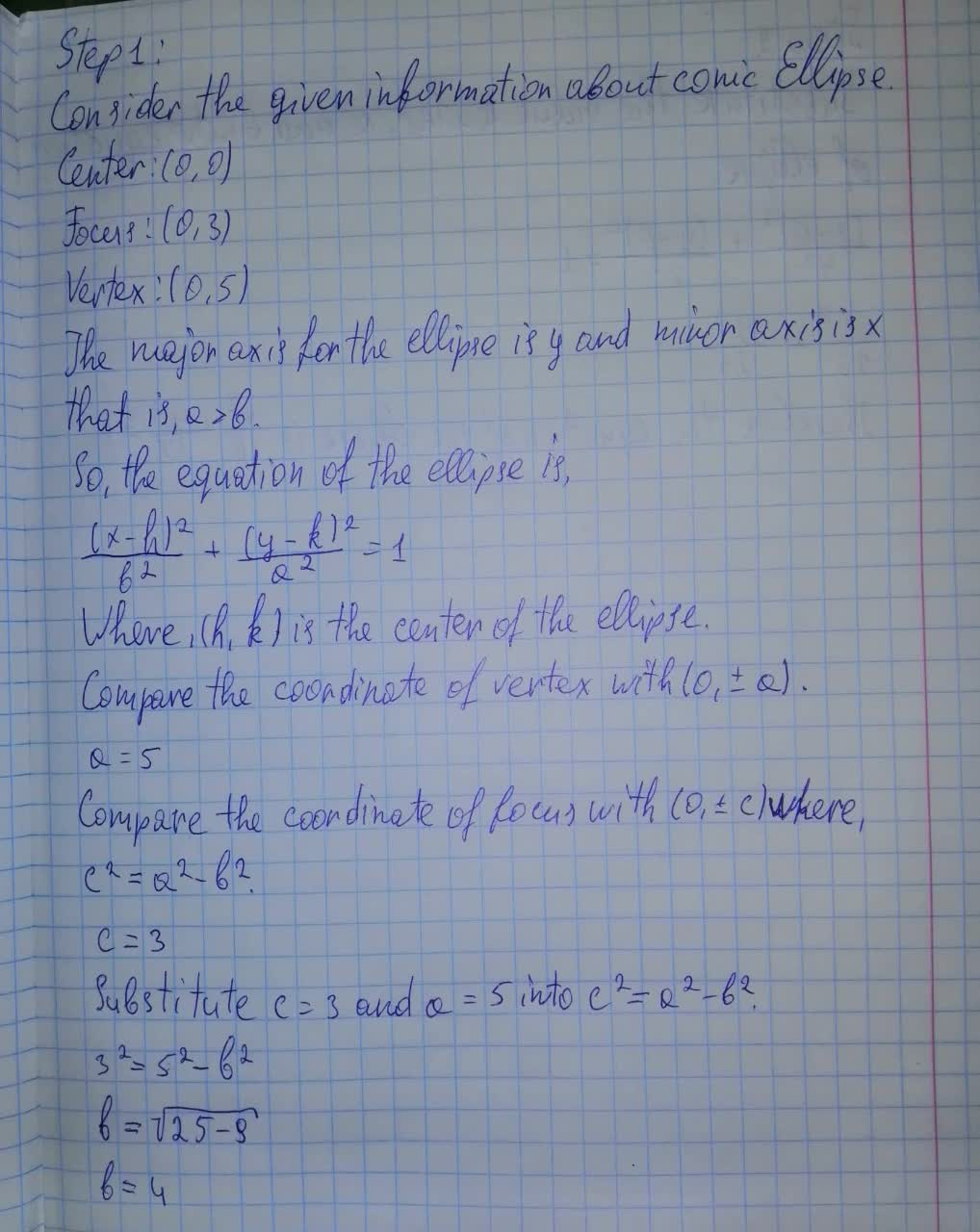# Find an equation of the conic described.Graph the equation.Ellipse; center at (0,0); focus at (0,3); vertex at (0, 5)nicekikah 2021-08-13 Answered
Find an equation of the conic described.Graph the equation.
Ellipse; center at (0,0); focus at (0,3); vertex at (0, 5)You can still ask an expert for help

• Questions are typically answered in as fast as 30 minutes

Solve your problem for the price of one coffee

• Math expert for every subject
• Pay only if we can solve itDerrick

The solution is written below###### Not exactly what you’re looking for?Vasquez

Step 1

Consider the given information about conic Ellipse.

Center: (0,0)

Focus: (0,3)

Vertex: (0,5)

The major axis for the ellipse is y and minor axis is x that is, a>b

So, the equation of the ellipse is,

$\frac{\left(x-h{\right)}^{2}}{{b}^{2}}+\frac{\left(y-k{\right)}^{2}}{{a}^{2}}=1$

Where, (h,k) is the center of the ellipse

Compare the coordinate of vertex with $\left(0,±a\right)$

a=5

Compare the coordinate of focus with $\left(0,±c\right)$ where,

${c}^{2}={a}^{2}-{b}^{2}$

c=3

Substitute c=3 and a=5 into ${c}^{2}={a}^{2}-{b}^{2}$

${3}^{2}={5}^{2}-{b}^{2}$

$b=\sqrt{25-9}$

b=4

Step 2

Substitute the value center, a and b into equation of ellipse

$\frac{\left(x-0{\right)}^{2}}{{4}^{2}}+\frac{\left(y-0{\right)}^{2}}{{5}^{2}}=1$

$\frac{{x}^{2}}{16}+\frac{{y}^{2}}{25}=1$

therefore, the equation of the ellipse is $\frac{{x}^{2}}{16}+\frac{{y}^{2}}{25}=1$